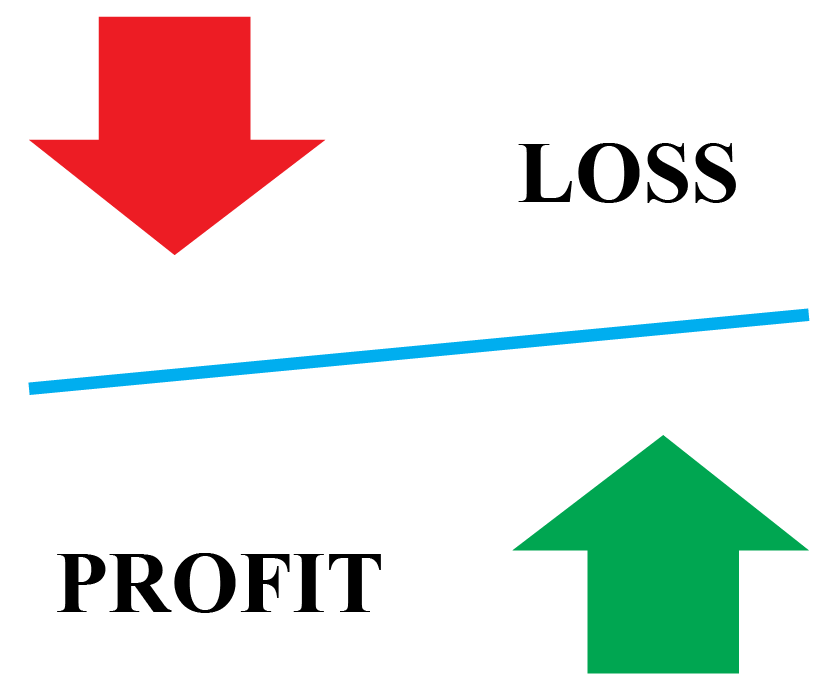# Profit and Loss

In any business transaction, we either make a profit or incur a loss. For running a business successfully or striking a sweet deal, understanding profit and loss is very important. And that is what we will be exploring on this page.

In this mini-lesson, let us learn about the selling price and cost price, how to calculate profit, to apply the profit and loss formula in our real-life problems.You can check out the interactive examples to know more about the lesson and try your hand at solving a few interesting practice questions at the end of the page.

## Lesson Plan

 1 What Is Profit and Loss? 2 Tips and Tricks 3 Important Notes on Profit and Loss 4 Solved Examples on Profit and Loss 5 Interactive Questions on Profit and Loss

## What Is Profit and Loss?

When a person buys or purchases an article for a certain price and then sells it for a different price, he makes a profit or incurs a loss.

Cost Price Selling Price
The price at which an article is purchased (CP) The price at which an article is sold (SP)
Profit Loss
Selling Price > Cost price Selling Price <  Cost price

Example

A book bought at $35 sold at$40.  $35$40 Profit of $5 ## What Is Cost Price, Marked Price, and Selling Price? In order to cope with the competition in business and to boost the sale of goods, shopkeepers offer discounts to customers.### Discount The rebate or the offer given by the shopkeepers to lure the customers is called a discount. 40$$\%$$ discount means for every$100 SP, the rebate offered is $40. The customer can pay only$60 for every $100.$40 is waived off.

 Discount = Marked Price - Selling Price

 CP = $300 SP =$280 LOSS = $20 FRACTION = $$\dfrac{20}{300} = \dfrac{1}{15}$$ ### Profit Formula  $$\text{Profit}\% = (\dfrac{\text{Profit}}{\text{CP}}) \times 100$$ CP SP PROFIT/LOSS$450 $495 Profit =$45
FRACTION $$\%$$
$$\dfrac{45}{450} = \dfrac{1}{10}$$ Profit %$$=\dfrac{1}{10}\times 100 = 10\%$$

### Loss Formula

 $$\text{Loss}\% = (\dfrac{\text{Loss}}{\text{CP}}) \times 100$$
CP SP PROFIT/LOSS
$400 350$ Loss = 50$FRACTION $$\%$$ $$\dfrac{50}{400}= \dfrac{1}{8}$$ Loss %$$=\dfrac{1}{8}\times 100 = 12 \dfrac{1}{2}\%$$ Example If SP =$800, MP = $1000 and SP =$900, then let's find the discount$$\%$$ and the profit$$\%$$ using the profit formula.

\begin{align}\text{Discount}\\ &= \text{MP - SP}\\ &= 1000 - 900\\ &= 100\end{align}

\begin{align}\text{Discount} \%\\\\ &= \dfrac{\text{discount}}{\text{MP}}\times 100\%\\\\ &= \dfrac{100}{1000}\times 100\%\\\\&=10\%\end{align}

\begin{align}\text{Profit}\\&= \text{SP - CP}\\&= 900 - 800\\ &= 100\end{align}

\begin{align}\text{Profit}\%\\\\&=\dfrac{\text{profit}}{\text{CP}} \times 100\%\\\\&=\dfrac{100}{800} \times 100\%\\\\&=12 \dfrac{1}{2}\%\end{align}

More Important Topics
Numbers
Algebra
Geometry
Measurement
Money
Data
Trigonometry
Calculus